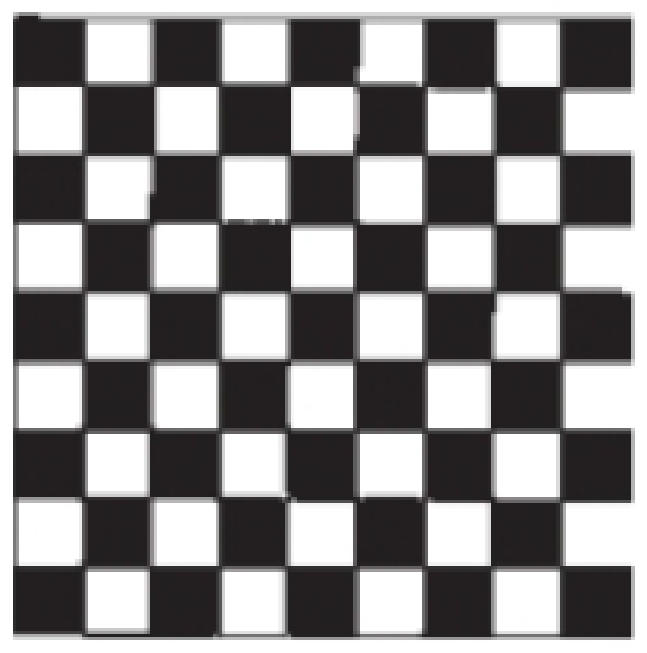Chapter 12, Problem 2PS

Chapter
Section
Textbook Problem

Outline a two-dimensional unit cell for the pattern shown here. If the black squares are labeled A and the white squares are B, what is the simplest formula for a “compound” based on this pattern?Interpretation Introduction

Interpretation:

The simplest formula of the compound has to be identified.

Explanation

According to the picture, we consider the unit pattern as combination of two molecules,

Formulaofthepattern=2Ablack squares+2 Bwhite squarestherefore,Formulaofthepattern

Still sussing out bartleby?

Check out a sample textbook solution.

See a sample solution

The Solution to Your Study Problems

Bartleby provides explanations to thousands of textbook problems written by our experts, many with advanced degrees!

Get Started